# Properties ofof Quadratic Functions in Properties Quadratic 2

• Slides: 50Properties ofof Quadratic Functions in Properties Quadratic 2 -2 Standard Form Functions in Standard Form Warm Up Lesson Presentation Lesson Quiz Holt. Mc. Dougal Algebra 2 Holt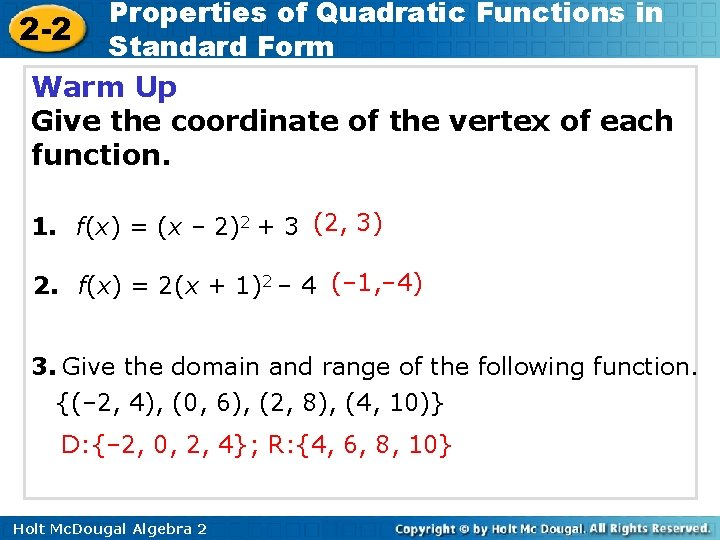Properties of Quadratic Functions in 2 -2 Standard Form Warm Up Give the coordinate of the vertex of each function. 1. f(x) = (x – 2)2 + 3 (2, 3) 2. f(x) = 2(x + 1)2 – 4 (– 1, – 4) 3. Give the domain and range of the following function. {(– 2, 4), (0, 6), (2, 8), (4, 10)} D: {– 2, 0, 2, 4}; R: {4, 6, 8, 10} Holt Mc. Dougal Algebra 2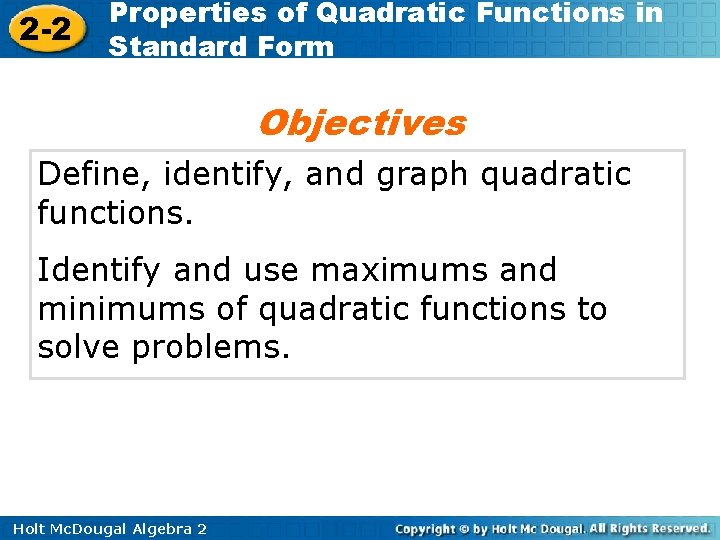2 -2 Properties of Quadratic Functions in Standard Form Objectives Define, identify, and graph quadratic functions. Identify and use maximums and minimums of quadratic functions to solve problems. Holt Mc. Dougal Algebra 22 -2 Properties of Quadratic Functions in Standard Form Vocabulary axis of symmetry standard form minimum value maximum value Holt Mc. Dougal Algebra 22 -2 Properties of Quadratic Functions in Standard Form When you transformed quadratic functions in the previous lesson, you saw that reflecting the parent function across the y-axis results in the same function. Holt Mc. Dougal Algebra 22 -2 Properties of Quadratic Functions in Standard Form This shows that parabolas are symmetric curves. The axis of symmetry is the line through the vertex of a parabola that divides the parabola into two congruent halves. Holt Mc. Dougal Algebra 2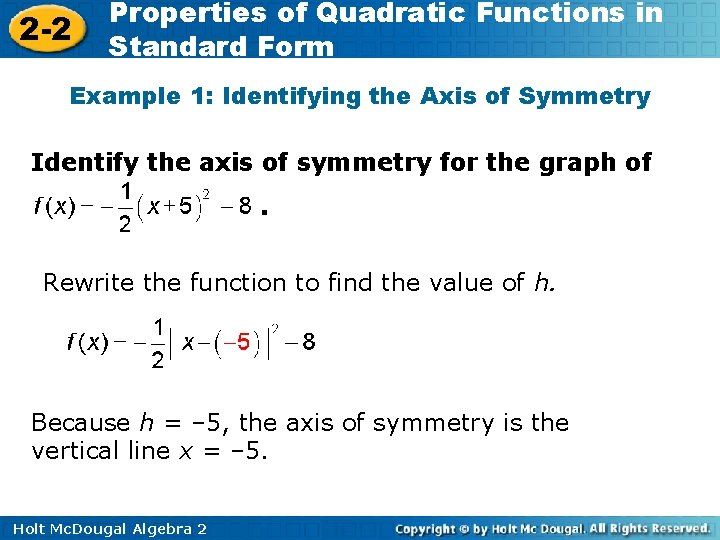2 -2 Properties of Quadratic Functions in Standard Form Example 1: Identifying the Axis of Symmetry Identify the axis of symmetry for the graph of. Rewrite the function to find the value of h. Because h = – 5, the axis of symmetry is the vertical line x = – 5. Holt Mc. Dougal Algebra 22 -2 Properties of Quadratic Functions in Standard Form Example 1 Continued Check Analyze the graph on a graphing calculator. The parabola is symmetric about the vertical line x = – 5. Holt Mc. Dougal Algebra 2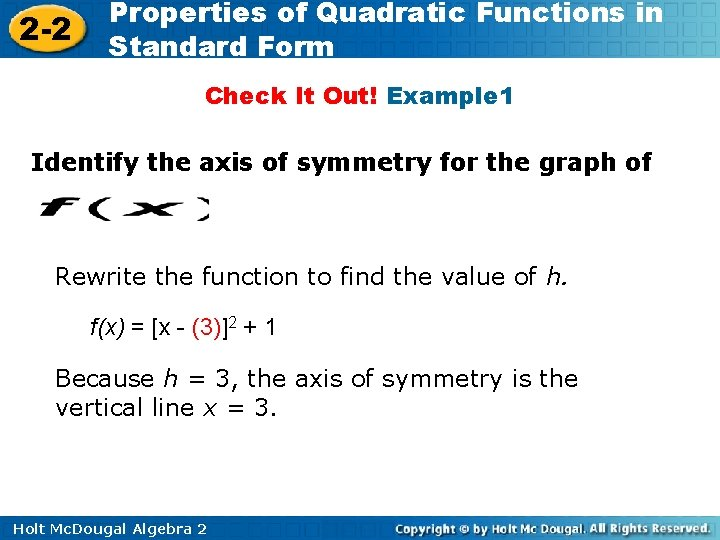2 -2 Properties of Quadratic Functions in Standard Form Check It Out! Example 1 Identify the axis of symmetry for the graph of Rewrite the function to find the value of h. f(x) = [x - (3)]2 + 1 Because h = 3, the axis of symmetry is the vertical line x = 3. Holt Mc. Dougal Algebra 22 -2 Properties of Quadratic Functions in Standard Form Check It Out! Example 1 Continued Check Analyze the graph on a graphing calculator. The parabola is symmetric about the vertical line x = 3. Holt Mc. Dougal Algebra 2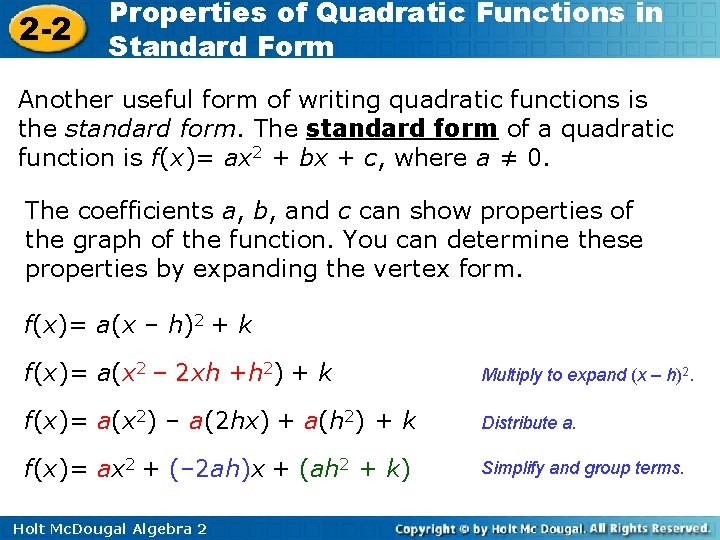2 -2 Properties of Quadratic Functions in Standard Form Another useful form of writing quadratic functions is the standard form. The standard form of a quadratic function is f(x)= ax 2 + bx + c, where a ≠ 0. The coefficients a, b, and c can show properties of the graph of the function. You can determine these properties by expanding the vertex form. f(x)= a(x – h)2 + k f(x)= a(x 2 – 2 xh +h 2) + k Multiply to expand (x – h)2. f(x)= a(x 2) – a(2 hx) + a(h 2) + k Distribute a. f(x)= ax 2 + (– 2 ah)x + (ah 2 + k) Simplify and group terms. Holt Mc. Dougal Algebra 22 -2 a=a Properties of Quadratic Functions in Standard Form a in standard form is the same as in vertex form. It indicates whether a reflection and/or vertical stretch or compression has been applied. Holt Mc. Dougal Algebra 22 -2 Properties of Quadratic Functions in Standard Form Solving for h gives. Therefore, b =– 2 ah the axis of symmetry, x = h, for a quadratic function in standard form is. Holt Mc. Dougal Algebra 2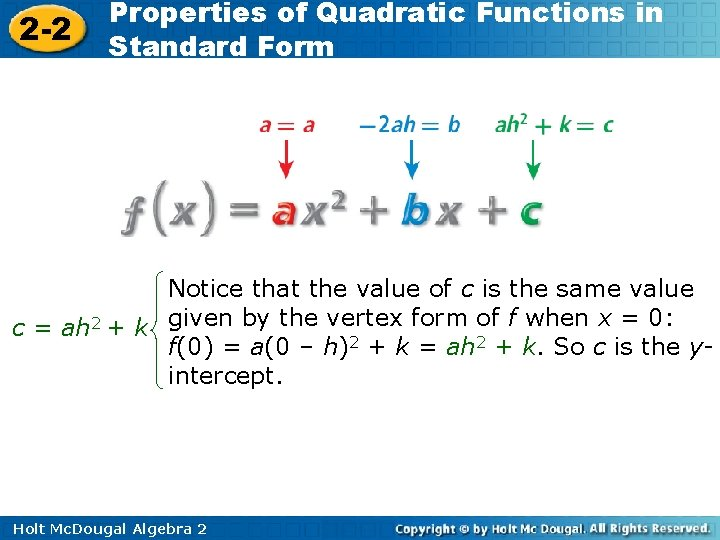2 -2 Properties of Quadratic Functions in Standard Form Notice that the value of c is the same value form of f when x = 0: c = ah 2 + k given by the vertex f(0) = a(0 – h)2 + k = ah 2 + k. So c is the yintercept. Holt Mc. Dougal Algebra 22 -2 Properties of Quadratic Functions in Standard Form These properties can be generalized to help you graph quadratic functions. Holt Mc. Dougal Algebra 2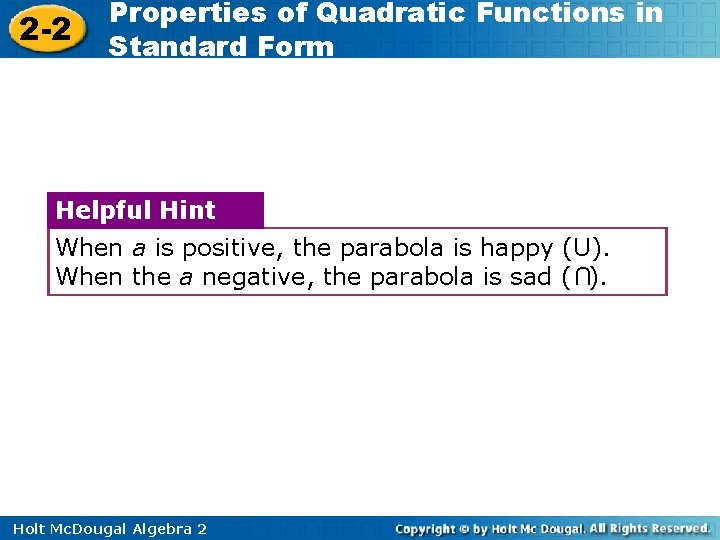2 -2 Properties of Quadratic Functions in Standard Form Helpful Hint When a is positive, the parabola is happy (U). When the a negative, the parabola is sad ( ). U Holt Mc. Dougal Algebra 2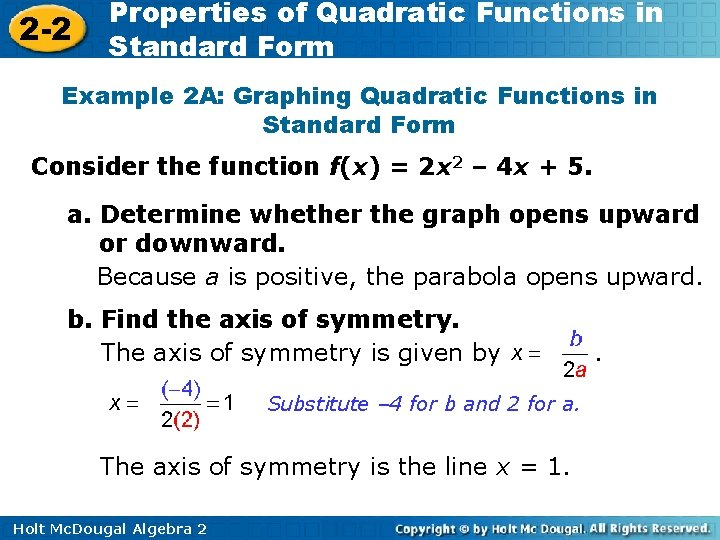2 -2 Properties of Quadratic Functions in Standard Form Example 2 A: Graphing Quadratic Functions in Standard Form Consider the function f(x) = 2 x 2 – 4 x + 5. a. Determine whether the graph opens upward or downward. Because a is positive, the parabola opens upward. b. Find the axis of symmetry. The axis of symmetry is given by Substitute – 4 for b and 2 for a. The axis of symmetry is the line x = 1. Holt Mc. Dougal Algebra 2 .2 -2 Properties of Quadratic Functions in Standard Form Example 2 A: Graphing Quadratic Functions in Standard Form Consider the function f(x) = 2 x 2 – 4 x + 5. c. Find the vertex. The vertex lies on the axis of symmetry, so the x-coordinate is 1. The y-coordinate is the value of the function at this x-value, or f(1) = 2(1)2 – 4(1) + 5 = 3 The vertex is (1, 3). d. Find the y-intercept. Because c = 5, the intercept is 5. Holt Mc. Dougal Algebra 2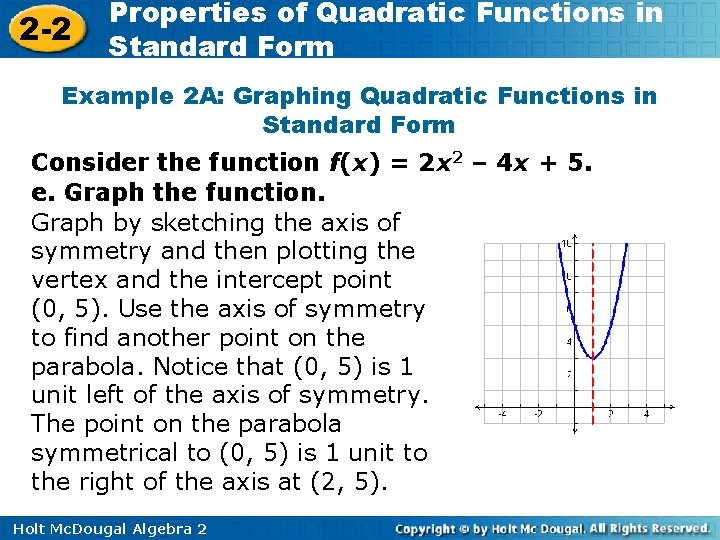2 -2 Properties of Quadratic Functions in Standard Form Example 2 A: Graphing Quadratic Functions in Standard Form Consider the function f(x) = 2 x 2 – 4 x + 5. e. Graph the function. Graph by sketching the axis of symmetry and then plotting the vertex and the intercept point (0, 5). Use the axis of symmetry to find another point on the parabola. Notice that (0, 5) is 1 unit left of the axis of symmetry. The point on the parabola symmetrical to (0, 5) is 1 unit to the right of the axis at (2, 5). Holt Mc. Dougal Algebra 2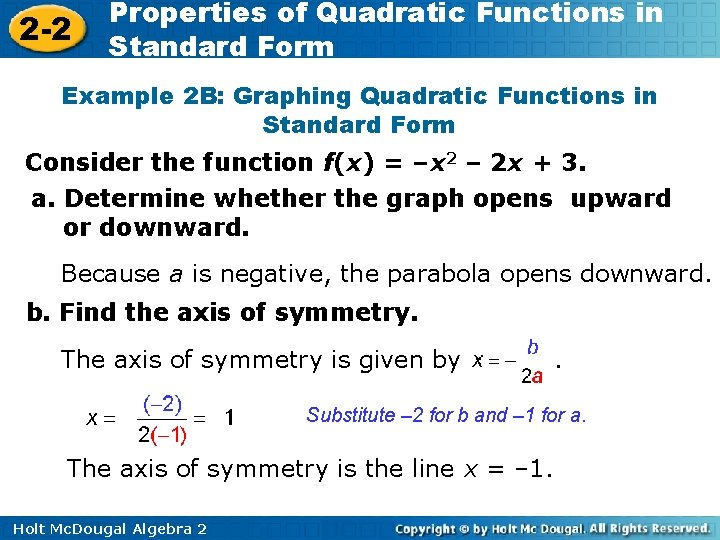2 -2 Properties of Quadratic Functions in Standard Form Example 2 B: Graphing Quadratic Functions in Standard Form Consider the function f(x) = –x 2 – 2 x + 3. a. Determine whether the graph opens upward or downward. Because a is negative, the parabola opens downward. b. Find the axis of symmetry. The axis of symmetry is given by . Substitute – 2 for b and – 1 for a. The axis of symmetry is the line x = – 1. Holt Mc. Dougal Algebra 2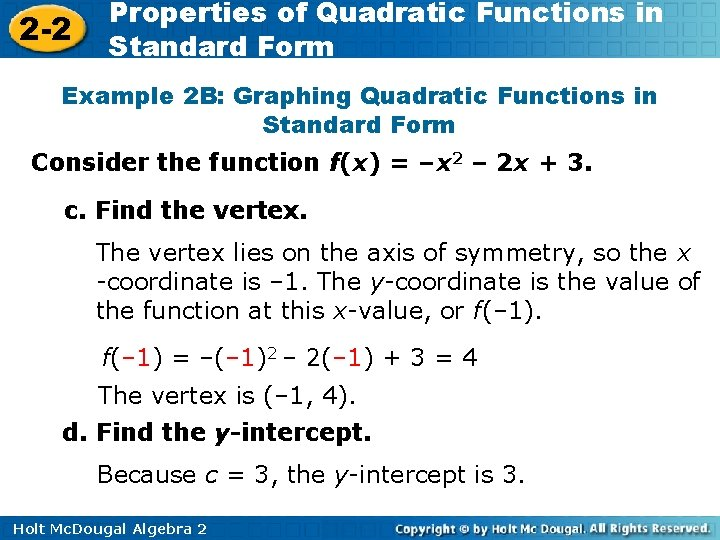2 -2 Properties of Quadratic Functions in Standard Form Example 2 B: Graphing Quadratic Functions in Standard Form Consider the function f(x) = –x 2 – 2 x + 3. c. Find the vertex. The vertex lies on the axis of symmetry, so the x -coordinate is – 1. The y-coordinate is the value of the function at this x-value, or f(– 1) = –(– 1)2 – 2(– 1) + 3 = 4 The vertex is (– 1, 4). d. Find the y-intercept. Because c = 3, the y-intercept is 3. Holt Mc. Dougal Algebra 2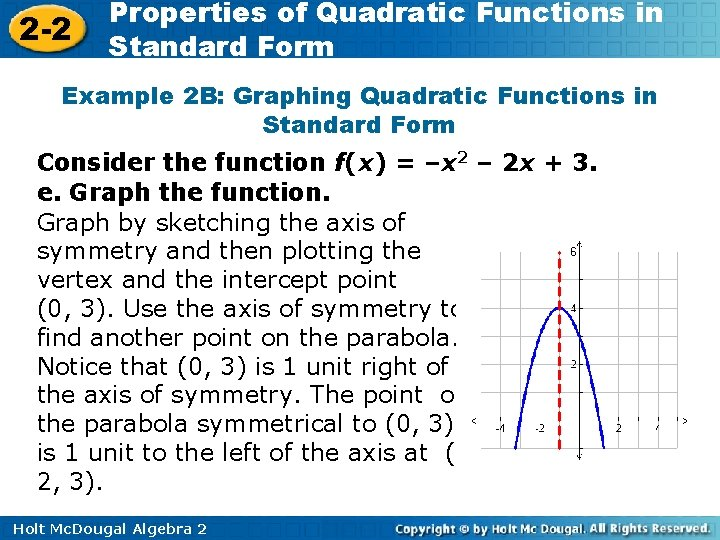2 -2 Properties of Quadratic Functions in Standard Form Example 2 B: Graphing Quadratic Functions in Standard Form Consider the function f(x) = –x 2 – 2 x + 3. e. Graph the function. Graph by sketching the axis of symmetry and then plotting the vertex and the intercept point (0, 3). Use the axis of symmetry to find another point on the parabola. Notice that (0, 3) is 1 unit right of the axis of symmetry. The point on the parabola symmetrical to (0, 3) is 1 unit to the left of the axis at (– 2, 3). Holt Mc. Dougal Algebra 22 -2 Properties of Quadratic Functions in Standard Form Check It Out! Example 2 a For the function, (a) determine whether the graph opens upward or downward, (b) find the axis of symmetry, (c) find the vertex, (d) find the y-intercept, and (e) graph the function. f(x)= – 2 x 2 – 4 x a. Because a is negative, the parabola opens downward. b. The axis of symmetry is given by . Substitute – 4 for b and – 2 for a. The axis of symmetry is the line x = – 1. Holt Mc. Dougal Algebra 2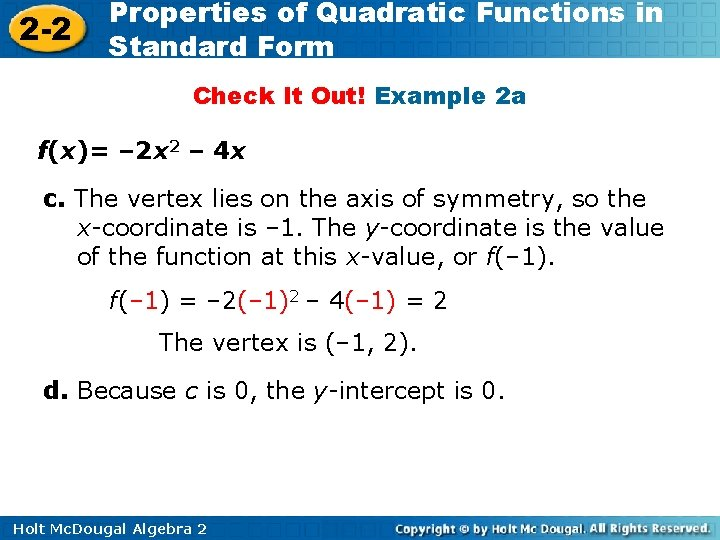2 -2 Properties of Quadratic Functions in Standard Form Check It Out! Example 2 a f(x)= – 2 x 2 – 4 x c. The vertex lies on the axis of symmetry, so the x-coordinate is – 1. The y-coordinate is the value of the function at this x-value, or f(– 1) = – 2(– 1)2 – 4(– 1) = 2 The vertex is (– 1, 2). d. Because c is 0, the y-intercept is 0. Holt Mc. Dougal Algebra 22 -2 Properties of Quadratic Functions in Standard Form Check It Out! Example 2 a f(x)= – 2 x 2 – 4 x e. Graph the function. Graph by sketching the axis of symmetry and then plotting the vertex and the intercept point (0, 0). Use the axis of symmetry to find another point on the parabola. Notice that (0, 0) is 1 unit right of the axis of symmetry. The point on the parabola symmetrical to (0, 0) is 1 unit to the left of the axis at (0, – 2). Holt Mc. Dougal Algebra 22 -2 Properties of Quadratic Functions in Standard Form Check It Out! Example 2 b For the function, (a) determine whether the graph opens upward or downward, (b) find the axis of symmetry, (c) find the vertex, (d) find the y-intercept, and (e) graph the function. g(x)= x 2 + 3 x – 1. a. Because a is positive, the parabola opens upward. b. The axis of symmetry is given by . Substitute 3 for b and 1 for a. The axis of symmetry is the line Holt Mc. Dougal Algebra 2 .2 -2 Properties of Quadratic Functions in Standard Form Check It Out! Example 2 b g(x)= x 2 + 3 x – 1 c. The vertex lies on the axis of symmetry, so the x-coordinate is. The y-coordinate is the value of the function at this x-value, or f( )=( The vertex is ( )2 + 3( , )– 1= ). d. Because c = – 1, the intercept is – 1. Holt Mc. Dougal Algebra 22 -2 Properties of Quadratic Functions in Standard Form Check It Out! Example 2 e. Graph the function. Graph by sketching the axis of symmetry and then plotting the vertex and the intercept point (0, – 1). Use the axis of symmetry to find another point on the parabola. Notice that (0, – 1) is 1. 5 units right of the axis of symmetry. The point on the parabola symmetrical to (0, – 1) is 1. 5 units to the left of the axis at (– 3, – 1). Holt Mc. Dougal Algebra 22 -2 Properties of Quadratic Functions in Standard Form Substituting any real value of x into a quadratic equation results in a real number. Therefore, the domain of any quadratic function is all real numbers. The range of a quadratic function depends on its vertex and the direction that the parabola opens. Holt Mc. Dougal Algebra 22 -2 Properties of Quadratic Functions in Standard Form Holt Mc. Dougal Algebra 2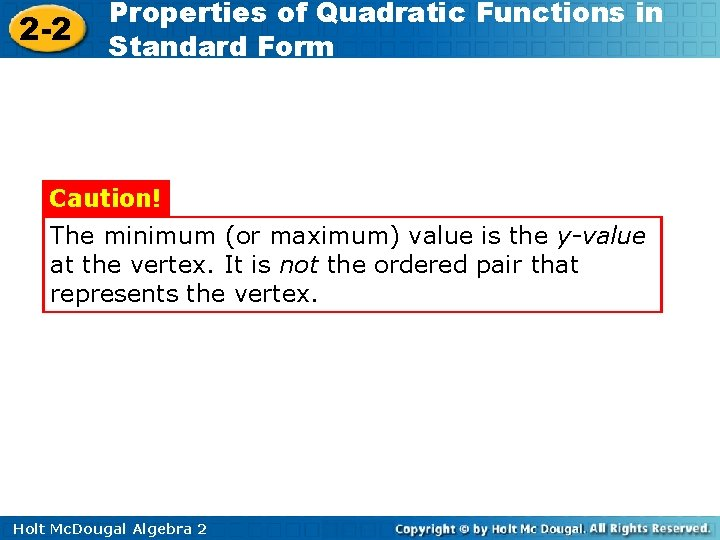2 -2 Properties of Quadratic Functions in Standard Form Caution! The minimum (or maximum) value is the y-value at the vertex. It is not the ordered pair that represents the vertex. Holt Mc. Dougal Algebra 2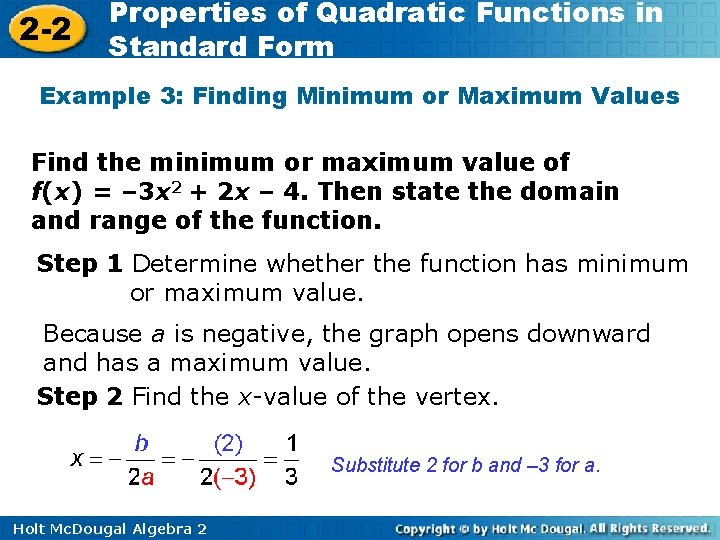2 -2 Properties of Quadratic Functions in Standard Form Example 3: Finding Minimum or Maximum Values Find the minimum or maximum value of f(x) = – 3 x 2 + 2 x – 4. Then state the domain and range of the function. Step 1 Determine whether the function has minimum or maximum value. Because a is negative, the graph opens downward and has a maximum value. Step 2 Find the x-value of the vertex. Substitute 2 for b and – 3 for a. Holt Mc. Dougal Algebra 2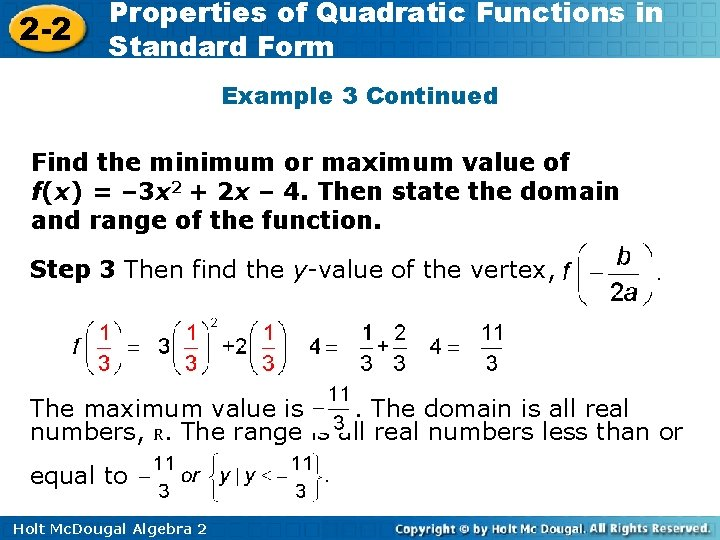2 -2 Properties of Quadratic Functions in Standard Form Example 3 Continued Find the minimum or maximum value of f(x) = – 3 x 2 + 2 x – 4. Then state the domain and range of the function. Step 3 Then find the y-value of the vertex, The maximum value is. The domain is all real numbers, R. The range is all real numbers less than or equal to Holt Mc. Dougal Algebra 2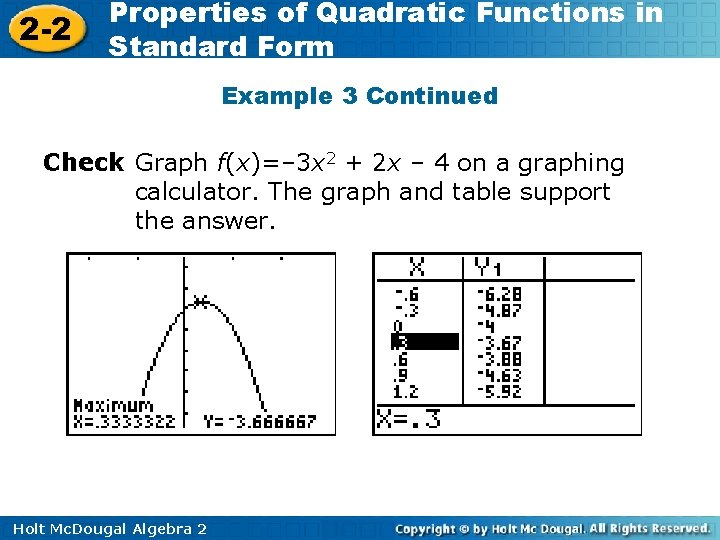2 -2 Properties of Quadratic Functions in Standard Form Example 3 Continued Check Graph f(x)=– 3 x 2 + 2 x – 4 on a graphing calculator. The graph and table support the answer. Holt Mc. Dougal Algebra 2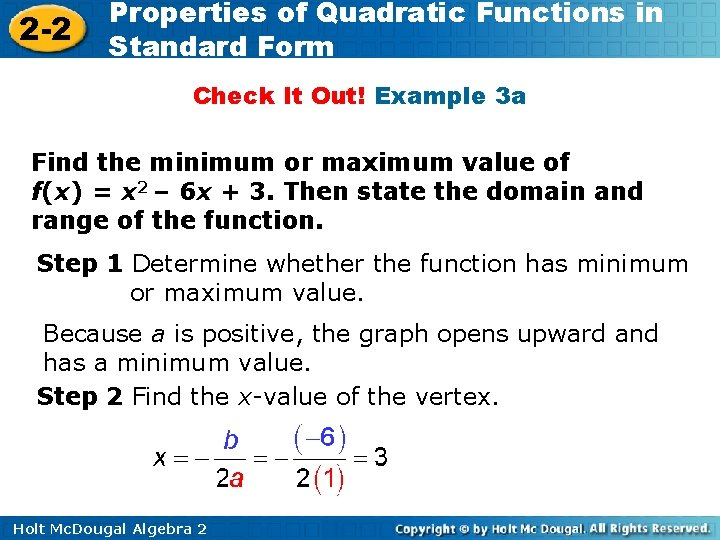2 -2 Properties of Quadratic Functions in Standard Form Check It Out! Example 3 a Find the minimum or maximum value of f(x) = x 2 – 6 x + 3. Then state the domain and range of the function. Step 1 Determine whether the function has minimum or maximum value. Because a is positive, the graph opens upward and has a minimum value. Step 2 Find the x-value of the vertex. Holt Mc. Dougal Algebra 2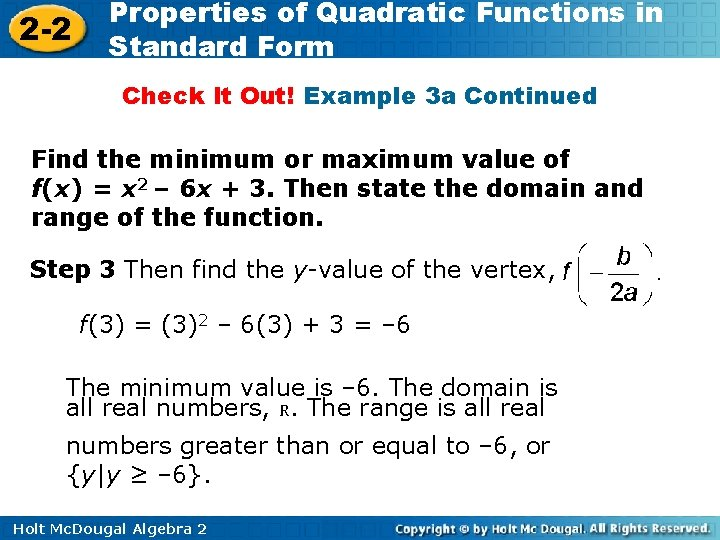2 -2 Properties of Quadratic Functions in Standard Form Check It Out! Example 3 a Continued Find the minimum or maximum value of f(x) = x 2 – 6 x + 3. Then state the domain and range of the function. Step 3 Then find the y-value of the vertex, f(3) = (3)2 – 6(3) + 3 = – 6 The minimum value is – 6. The domain is all real numbers, R. The range is all real numbers greater than or equal to – 6, or {y|y ≥ – 6}. Holt Mc. Dougal Algebra 22 -2 Properties of Quadratic Functions in Standard Form Check It Out! Example 3 a Continued Check Graph f(x)=x 2 – 6 x + 3 on a graphing calculator. The graph and table support the answer. Holt Mc. Dougal Algebra 2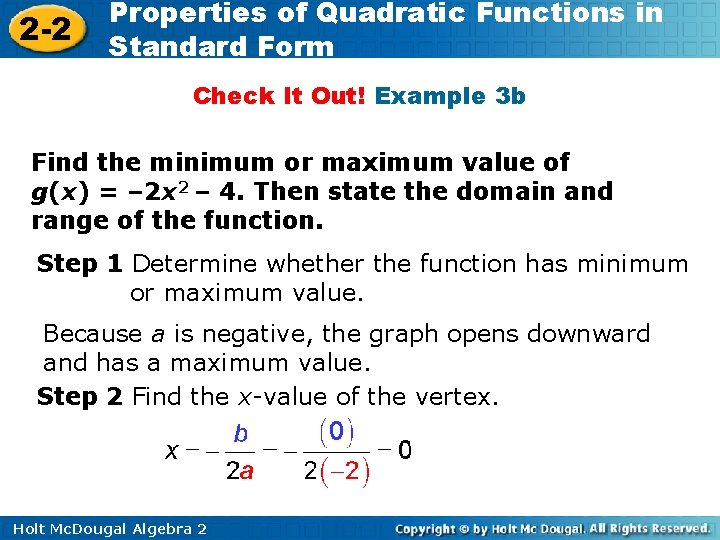2 -2 Properties of Quadratic Functions in Standard Form Check It Out! Example 3 b Find the minimum or maximum value of g(x) = – 2 x 2 – 4. Then state the domain and range of the function. Step 1 Determine whether the function has minimum or maximum value. Because a is negative, the graph opens downward and has a maximum value. Step 2 Find the x-value of the vertex. Holt Mc. Dougal Algebra 22 -2 Properties of Quadratic Functions in Standard Form Check It Out! Example 3 b Continued Find the minimum or maximum value of g(x) = – 2 x 2 – 4. Then state the domain and range of the function. Step 3 Then find the y-value of the vertex, f(0) = – 2(0)2 – 4 = – 4 The maximum value is – 4. The domain is all real numbers, R. The range is all real numbers less than or equal to – 4, or {y|y ≤ – 4}. Holt Mc. Dougal Algebra 22 -2 Properties of Quadratic Functions in Standard Form Check It Out! Example 3 b Continued Check Graph f(x)=– 2 x 2 – 4 on a graphing calculator. The graph and table support the answer. Holt Mc. Dougal Algebra 2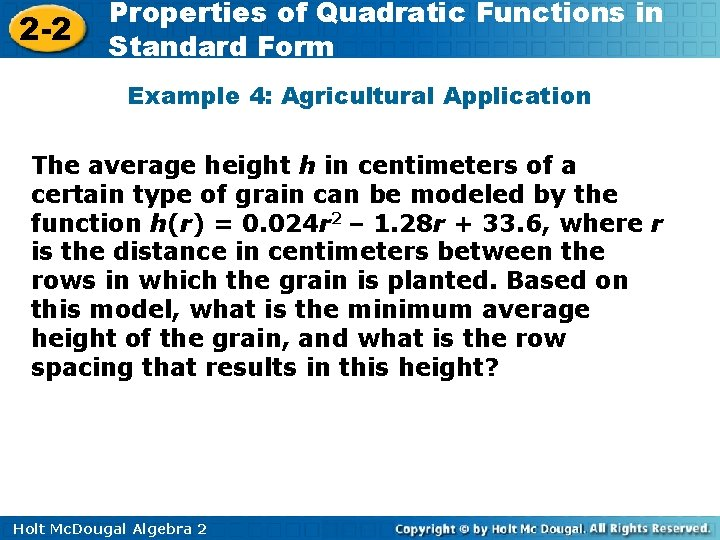2 -2 Properties of Quadratic Functions in Standard Form Example 4: Agricultural Application The average height h in centimeters of a certain type of grain can be modeled by the function h(r) = 0. 024 r 2 – 1. 28 r + 33. 6, where r is the distance in centimeters between the rows in which the grain is planted. Based on this model, what is the minimum average height of the grain, and what is the row spacing that results in this height? Holt Mc. Dougal Algebra 2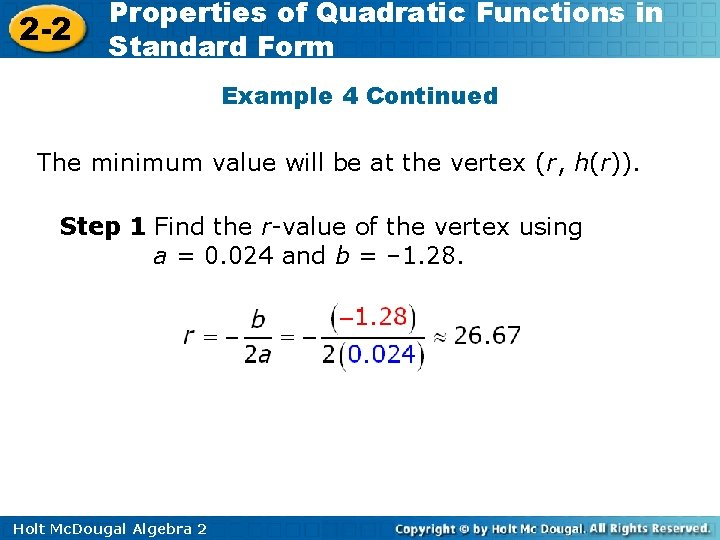2 -2 Properties of Quadratic Functions in Standard Form Example 4 Continued The minimum value will be at the vertex (r, h(r)). Step 1 Find the r-value of the vertex using a = 0. 024 and b = – 1. 28. Holt Mc. Dougal Algebra 2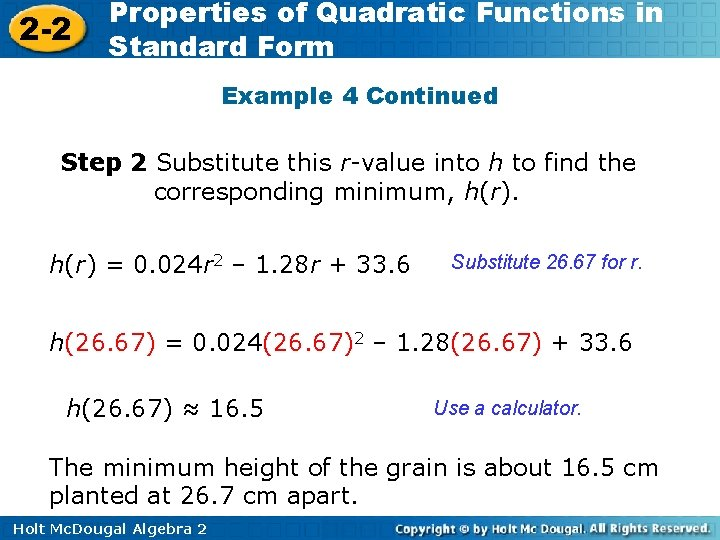2 -2 Properties of Quadratic Functions in Standard Form Example 4 Continued Step 2 Substitute this r-value into h to find the corresponding minimum, h(r) = 0. 024 r 2 – 1. 28 r + 33. 6 Substitute 26. 67 for r. h(26. 67) = 0. 024(26. 67)2 – 1. 28(26. 67) + 33. 6 h(26. 67) ≈ 16. 5 Use a calculator. The minimum height of the grain is about 16. 5 cm planted at 26. 7 cm apart. Holt Mc. Dougal Algebra 2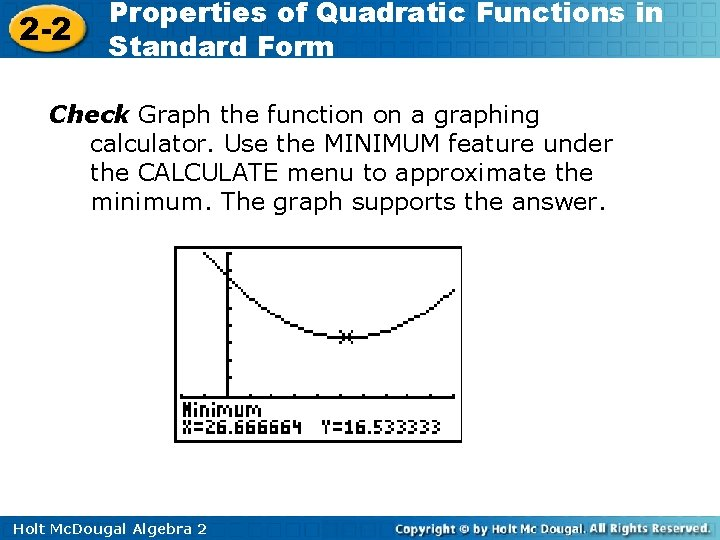2 -2 Properties of Quadratic Functions in Standard Form Check Graph the function on a graphing calculator. Use the MINIMUM feature under the CALCULATE menu to approximate the minimum. The graph supports the answer. Holt Mc. Dougal Algebra 2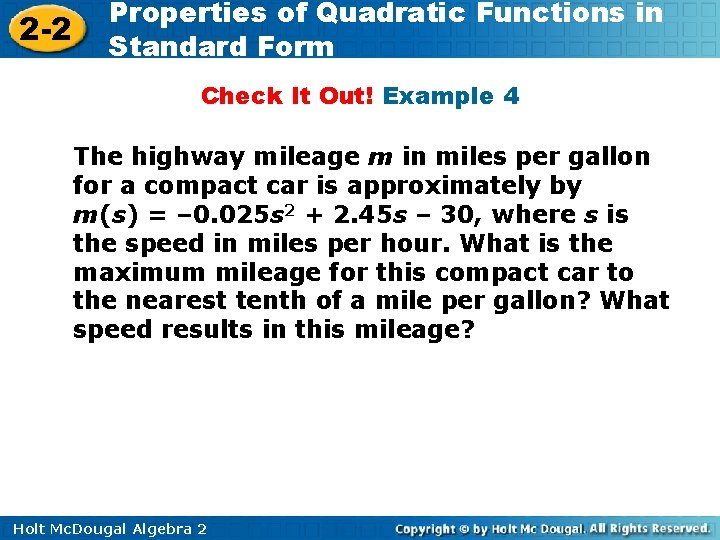2 -2 Properties of Quadratic Functions in Standard Form Check It Out! Example 4 The highway mileage m in miles per gallon for a compact car is approximately by m(s) = – 0. 025 s 2 + 2. 45 s – 30, where s is the speed in miles per hour. What is the maximum mileage for this compact car to the nearest tenth of a mile per gallon? What speed results in this mileage? Holt Mc. Dougal Algebra 2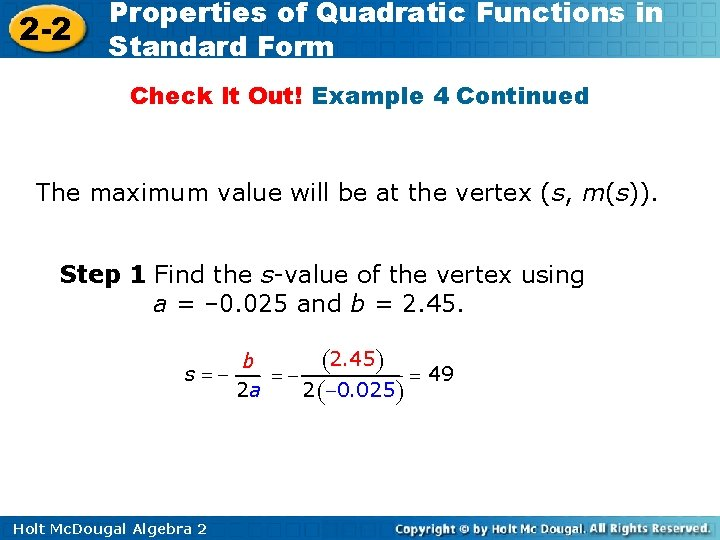2 -2 Properties of Quadratic Functions in Standard Form Check It Out! Example 4 Continued The maximum value will be at the vertex (s, m(s)). Step 1 Find the s-value of the vertex using a = – 0. 025 and b = 2. 45 ) ( b s === 49 2 a 2 (-0. 025) Holt Mc. Dougal Algebra 2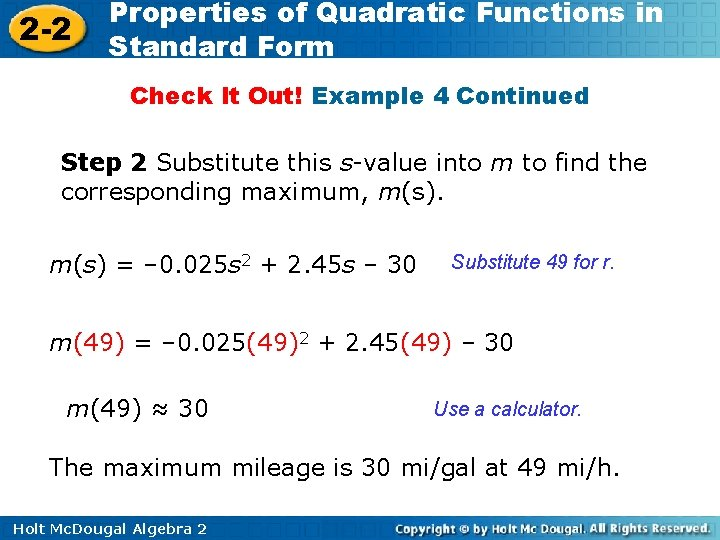2 -2 Properties of Quadratic Functions in Standard Form Check It Out! Example 4 Continued Step 2 Substitute this s-value into m to find the corresponding maximum, m(s) = – 0. 025 s 2 + 2. 45 s – 30 Substitute 49 for r. m(49) = – 0. 025(49)2 + 2. 45(49) – 30 m(49) ≈ 30 Use a calculator. The maximum mileage is 30 mi/gal at 49 mi/h. Holt Mc. Dougal Algebra 2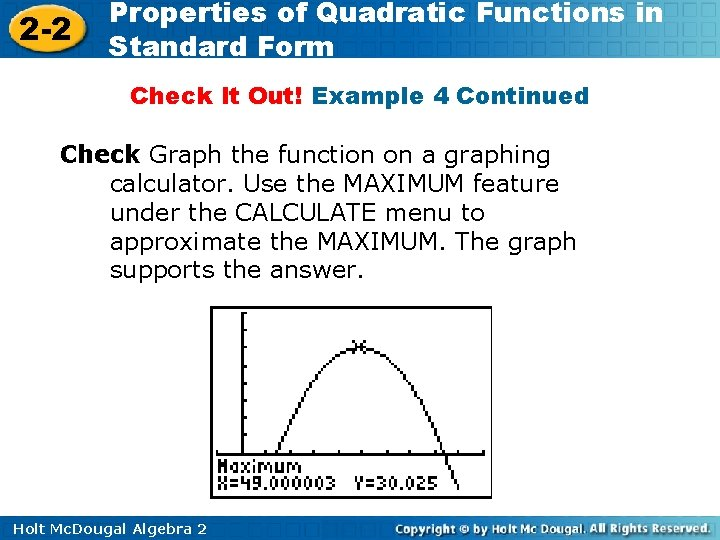2 -2 Properties of Quadratic Functions in Standard Form Check It Out! Example 4 Continued Check Graph the function on a graphing calculator. Use the MAXIMUM feature under the CALCULATE menu to approximate the MAXIMUM. The graph supports the answer. Holt Mc. Dougal Algebra 2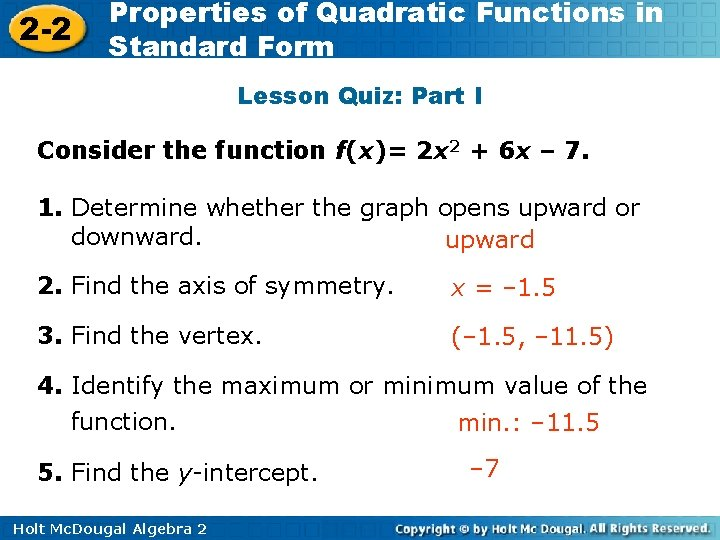2 -2 Properties of Quadratic Functions in Standard Form Lesson Quiz: Part I Consider the function f(x)= 2 x 2 + 6 x – 7. 1. Determine whether the graph opens upward or downward. upward 2. Find the axis of symmetry. x = – 1. 5 3. Find the vertex. (– 1. 5, – 11. 5) 4. Identify the maximum or minimum value of the function. min. : – 11. 5 5. Find the y-intercept. Holt Mc. Dougal Algebra 2 – 72 -2 Properties of Quadratic Functions in Standard Form Lesson Quiz: Part II Consider the function f(x)= 2 x 2 + 6 x – 7. 6. Graph the function. 7. Find the domain and range of the function. D: All real numbers; R {y|y ≥ – 11. 5} Holt Mc. Dougal Algebra 2